# 概述

STL(Standard Template Library,标准模板库)，是惠普实验室开发的一系列软件的统称。现在主要出现在 c++中，但是在引入 c++之前该技术已经存在很长时间了。

STL 从广义上分为: 容器(container) 算法(algorithm) 迭代器(iterator)。

STL(Standard Template Library)标准模板库,在我们 c++标准程序库中隶属于 STL 的占到了 80%以上。

# STL六大组件简介

STL提供了六大组件，彼此之间可以组合套用，这六大组件分别是:容器算法迭代器仿函数适配器（配接器）空间配置器

STL六大组件的交互关系，容器通过空间配置器取得数据存储空间，算法通过迭代器存储容器中的内容，仿函数可以协助算法完成不同的策略的变化，适配器可以修饰仿函数。

STL的优点很明显了

• STL 是 C++的一部分，因此不用额外安装什么，它被内建在你的编译器之内。
• STL 的一个重要特性是将数据和操作分离。数据由容器类别加以管理，操作则由可定制的算法定义。迭代器在两者之间充当“粘合剂”,以使算法可以和容器交互运作
• 程序员可以不用思考 STL 具体的实现过程，只要能够熟练使用 STL 就 OK 了。这样他们就可以把精力放在程序开发的别的方面。
• STL 具有高可重用性，高性能，高移植性，跨平台的优点。
• 高可重用性：STL 中几乎所有的代码都采用了模板类和模版函数的方式实现，这相比于传统的由函数和类组成的库来说提供了更好的代码重用机会。
• 高性能：如 map 可以高效地从十万条记录里面查找出指定的记录，因为 map 是采用红黑树的变体实现的。
• 高移植性：如在项目 A 上用 STL 编写的模块，可以直接移植到项目 B 上。

# 三大组件介绍

## 1. 容器

• 序列式容器强调值的排序，序列式容器中的每个元素均有固定的位置，除非用删除或插入的操作改变这个位置。Vector容器、Deque容器、List容器等。
• 关联式容器是非线性的树结构，更准确的说是二叉树结构。各元素之间没有严格的物理上的顺序关系，也就是说元素在容器中并没有保存元素置入容器时的逻辑顺序。关联式容器另一个显著特点是：在值中选择一个值作为关键字key，这个关键字对值起到索引的作用，方便查找。Set/multiset容器 Map/multimap容器

## 2. 算法

• 质变算法：是指运算过程中会更改区间内的元素的内容。例如拷贝，替换，删除等等
• 非质变算法：是指运算过程中不会更改区间内的元素内容，例如查找、计数、遍历、寻找极值等等

## 3. 迭代器

#define _CRT_SECURE_NO_WARNINGS
#include<iostream>
#include<vector>
#include<algorithm>
using namespace std;

//STL 中的容器 算法 迭代器
void test01(){
vector<int> v; //STL 中的标准容器之一 ：动态数组
v.push_back(1); //vector 容器提供的插入数据的方法
v.push_back(5);
v.push_back(3);
v.push_back(7);
//迭代器
vector<int>::iterator pStart = v.begin(); //vector 容器提供了 begin()方法 返回指向第一个元素的迭代器
vector<int>::iterator pEnd = v.end(); //vector 容器提供了 end()方法 返回指向最后一个元素下一个位置的迭代器
//通过迭代器遍历
while (pStart != pEnd){
cout << *pStart << " ";
pStart++;
}
cout << endl;
//算法 count 算法 用于统计元素的个数
int n = count(pStart, pEnd, 5);
cout << "n:" << n << endl;
}
//STL 容器不单单可以存储基础数据类型，也可以存储类对象
class Teacher
{
public:
Teacher(int age) :age(age){};
~Teacher(){};
public:
int age;
};
void test02(){
vector<Teacher> v; //存储 Teacher 类型数据的容器
Teacher t1(10), t2(20), t3(30);
v.push_back(t1);
v.push_back(t2);
v.push_back(t3);
vector<Teacher>::iterator pStart = v.begin();
vector<Teacher>::iterator pEnd = v.end();
//通过迭代器遍历
while (pStart != pEnd){
cout << pStart->age << " ";
pStart++;
}
cout << endl;
}
//存储 Teacher 类型指针
void test03(){
vector<Teacher*> v; //存储 Teacher 类型指针
Teacher* t1 = new Teacher(10);
Teacher* t2 = new Teacher(20);
Teacher* t3 = new Teacher(30);
v.push_back(t1);
v.push_back(t2);
v.push_back(t3);
//拿到容器迭代器
vector<Teacher*>::iterator pStart = v.begin();
vector<Teacher*>::iterator pEnd = v.end();
//通过迭代器遍历
while (pStart != pEnd){
cout << (*pStart)->age << " ";
pStart++;
}
cout << endl;
}
//容器嵌套容器 难点
void test04()
{
vector< vector<int> > v;
vector<int>v1;
vector<int>v2;
vector<int>v3;

for (int i = 0; i < 5;i++)
{
v1.push_back(i);
v2.push_back(i * 10);
v3.push_back(i * 100);
}
v.push_back(v1);
v.push_back(v2);
v.push_back(v3);

for (vector< vector<int> >::iterator it = v.begin(); it != v.end();it++)
{
for (vector<int>::iterator subIt = (*it).begin(); subIt != (*it).end(); subIt ++)
{
cout << *subIt << " ";
}
cout << endl;
}
}
int main(){
//test01();
//test02();
//test03();
test04();
system("pause");
return EXIT_SUCCESS;
}


# 常用容器

## 1. string容器

### string容器基本概念

C风格字符串(以空字符结尾的字符数组)太过复杂难于掌握，不适合大程序的开发，所以C++标准库定义了一种string类，定义在头文件<string>。
String和c风格字符串对比：

• Char*是一个指针，String是一个类
string封装了char*，管理这个字符串，是一个char*型的容器。
• String封装了很多实用的成员方法
查找find，拷贝copy，删除delete 替换replace，插入insert
• 不用考虑内存释放和越界
string管理char*所分配的内存。每一次string的复制，取值都由string类负责维护，不用担心复制越界和取值越界等。

### string容器常用操作

string 构造函数

string();//创建一个空的字符串 例如: string str;
string(const string& str);//使用一个string对象初始化另一个string对象
string(const char* s);//使用字符串s初始化
string(int n, char c);//使用n个字符c初始化


string基本赋值操作

string& operator=(const char* s);//char*类型字符串 赋值给当前的字符串
string& operator=(const string &s);//把字符串s赋给当前的字符串
string& operator=(char c);//字符赋值给当前的字符串
string& assign(const char *s);//把字符串s赋给当前的字符串
string& assign(const char *s, int n);//把字符串s的前n个字符赋给当前的字符串
string& assign(const string &s);//把字符串s赋给当前字符串
string& assign(int n, char c);//用n个字符c赋给当前字符串
string& assign(const string &s, int start, int n);//将s从start开始n个字符赋值给字符串


string存取字符操作

char& operator[](int n);//通过[]方式取字符
char& at(int n);//通过at方法获取字符


string拼接操作

string& operator+=(const string& str);//重载+=操作符
string& operator+=(const char* str);//重载+=操作符
string& operator+=(const char c);//重载+=操作符
string& append(const char *s);//把字符串s连接到当前字符串结尾
string& append(const char *s, int n);//把字符串s的前n个字符连接到当前字符串结尾
string& append(const string &s);//同operator+=()
string& append(const string &s, int pos, int n);//把字符串s中从pos开始的n个字符连接到当前字符串结尾
string& append(int n, char c);//在当前字符串结尾添加n个字符c


string查找和替换

int find(const string& str, int pos = 0) const; //查找str第一次出现位置,从pos开始查找
int find(const char* s, int pos = 0) const;  //查找s第一次出现位置,从pos开始查找
int find(const char* s, int pos, int n) const;  //从pos位置查找s的前n个字符第一次位置
int find(const char c, int pos = 0) const;  //查找字符c第一次出现位置
int rfind(const string& str, int pos = npos) const;//查找str最后一次位置,从pos开始查找
int rfind(const char* s, int pos = npos) const;//查找s最后一次出现位置,从pos开始查找
int rfind(const char* s, int pos, int n) const;//从pos查找s的前n个字符最后一次位置
int rfind(const char c, int pos = 0) const; //查找字符c最后一次出现位置
string& replace(int pos, int n, const string& str); //替换从pos开始n个字符为字符串str
string& replace(int pos, int n, const char* s); //替换从pos开始的n个字符为字符串s


string比较操作

/*
compare函数在>时返回 1，<时返回 -1，==时返回 0。

*/
int compare(const string &s) const;//与字符串s比较
int compare(const char *s) const;//与字符串s比较


string子串

string substr(int pos = 0, int n = npos) const;//返回由pos开始的n个字符组成的字符串


string插入和删除操作

string& insert(int pos, const char* s); //插入字符串
string& insert(int pos, const string& str); //插入字符串
string& insert(int pos, int n, char c);//在指定位置插入n个字符c
string& erase(int pos, int n = npos);//删除从Pos开始的n个字符


string和c-style字符串转换

//string 转 char*
string str = "it";
const char* cstr = str.c_str();
//char* 转 string
char* s = "it";
string str(s);


string s = "abcdefg";
char& a = s;
char& b = s;

a = '1';
b = '2';

cout << s << endl;
cout << (int*)s.c_str() << endl;

s = "pppppppppppppppppppppppp";

//a = '1';
//b = '2';

cout << s << endl;
cout << (int*)s.c_str() << endl;


## 2. vector容器

### vector容器基本概念

vector的数据安排以及操作方式，与array非常相似，两者的唯一差别在于空间的运用的灵活性。

Array是静态空间，一旦配置了就不能改变，要换大一点或者小一点的空间，可以，一切琐碎得由自己来，首先配置一块新的空间，然后将旧空间的数据搬往新空间，再释放原来的空间。

Vector是动态空间，随着元素的加入，它的内部机制会自动扩充空间以容纳新元素。因此vector的运用对于内存的合理利用与运用的灵活性有很大的帮助，我们再也不必害怕空间不足而一开始就要求一个大块头的array了。

Vector的实现技术，关键在于其对大小的控制以及重新配置时的数据移动效率，一旦vector旧空间满了，如果客户每新增一个元素，vector内部只是扩充一个元素的空间，实为不智，因为所谓的扩充空间(不论多大)，一如刚所说，是”配置新空间-数据移动-释放旧空间”的大工程,时间成本很高，应该加入某种未雨绸缪的考虑，稍后我们便可以看到vector的空间配置策略。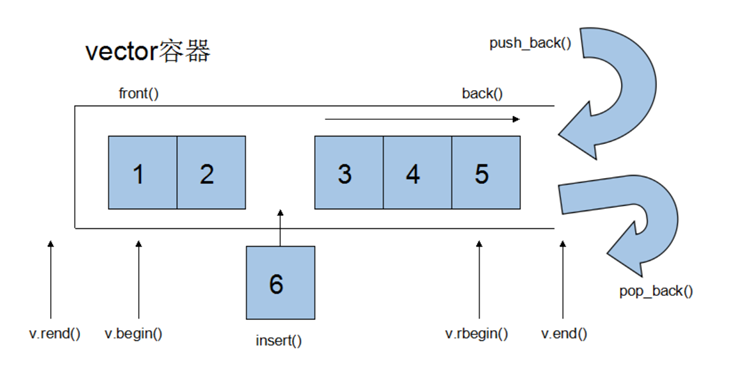### vector迭代器

Vector维护一个线性空间，所以不论元素的型别如何，普通指针都可以作为vector的迭代器，因为vector迭代器所需要的操作行为，如operaroe*, operator->, operator++, operator–, operator+, operator-, operator+=, operator-=, 普通指针天生具备。

Vector支持随机存取，而普通指针正有着这样的能力。所以vector提供的是随机访问迭代器(Random Access Iterators).

Vector<int>::iterator it1;
Vector<Teacher>::iterator it2;


it1的型别其实就是Int*,it2的型别其实就是Teacher*.

#define _CRT_SECURE_NO_WARNINGS
#include<iostream>
#include<vector>
using namespace std;

int main(){

vector<int> v;
for (int i = 0; i < 10;i ++){
v.push_back(i);
cout << v.capacity() << endl;  // v.capacity()容器的容量
}

system("pause");
return EXIT_SUCCESS;
}


### vector的数据结构

Vector所采用的数据结构非常简单，线性连续空间，它以两个迭代器_Myfirst和_Mylast分别指向配置得来的连续空间中目前已被使用的范围，并以迭代器_Myend指向整块连续内存空间的尾端。

### vector常用API操作

vector构造函数

vector<T> v; //采用模板实现类实现，默认构造函数
vector(v.begin(), v.end());//将v[begin(), end())区间中的元素拷贝给本身。
vector(n, elem);//构造函数将n个elem拷贝给本身。
vector(const vector &vec);//拷贝构造函数。

//例子 使用第二个构造函数 我们可以...
int arr[] = {2,3,4,1,9};
vector<int> v1(arr, arr + sizeof(arr) / sizeof(int));


vector常用赋值操作

assign(beg, end);//将[beg, end)区间中的数据拷贝赋值给本身。
assign(n, elem);//将n个elem拷贝赋值给本身。
vector& operator=(const vector  &vec);//重载等号操作符
swap(vec);// 将vec与本身的元素互换。


vector大小操作

size();//返回容器中元素的个数
empty();//判断容器是否为空
resize(int num);//重新指定容器的长度为num，若容器变长，则以默认值填充新位置。如果容器变短，则末尾超出容器长度的元素被删除。
resize(int num, elem);//重新指定容器的长度为num，若容器变长，则以elem值填充新位置。如果容器变短，则末尾超出容器长>度的元素被删除。
capacity();//容器的容量
reserve(int len);//容器预留len个元素长度，预留位置不初始化，元素不可访问。


vector数据存取操作

at(int idx); //返回索引idx所指的数据，如果idx越界，抛出out_of_range异常。
operator[];//返回索引idx所指的数据，越界时，运行直接报错
front();//返回容器中第一个数据元素
back();//返回容器中最后一个数据元素


vector插入和删除操作

insert(const_iterator pos, int count,ele);//迭代器指向位置pos插入count个元素ele.
push_back(ele); //尾部插入元素ele
pop_back();//删除最后一个元素
erase(const_iterator start, const_iterator end);//删除迭代器从start到end之间的元素
erase(const_iterator pos);//删除迭代器指向的元素
clear();//删除容器中所有元素


vector 小demo: 巧用swap，收缩内存空间

#define _CRT_SECURE_NO_WARNINGS
#include<iostream>
#include<vector>
using namespace std;

int main(){

vector<int> v;
for (int i = 0; i < 100000;i ++){
v.push_back(i);
}

cout << "capacity:" << v.capacity() << endl;
cout << "size:" << v.size() << endl;

//此时 通过resize改变容器大小
v.resize(10);

cout << "capacity:" << v.capacity() << endl;
cout << "size:" << v.size() << endl;

//容量没有改变
vector<int>(v).swap(v);

cout << "capacity:" << v.capacity() << endl;
cout << "size:" << v.size() << endl;

system("pause");
return EXIT_SUCCESS;
}


reserve预留空间

#define _CRT_SECURE_NO_WARNINGS
#include<iostream>
#include<vector>
using namespace std;

int main(){

vector<int> v;

//预先开辟空间
v.reserve(100000);

int* pStart = NULL;
int count = 0;
for (int i = 0; i < 100000;i ++){
v.push_back(i);
if (pStart != &v){
pStart = &v;
count++;
}
}

cout << "count:" << count << endl;

system("pause");
return EXIT_SUCCESS;
}


## 3. deque容器

### deque容器基本概念

Vector容器是单向开口的连续内存空间，deque则是一种双向开口的连续线性空间。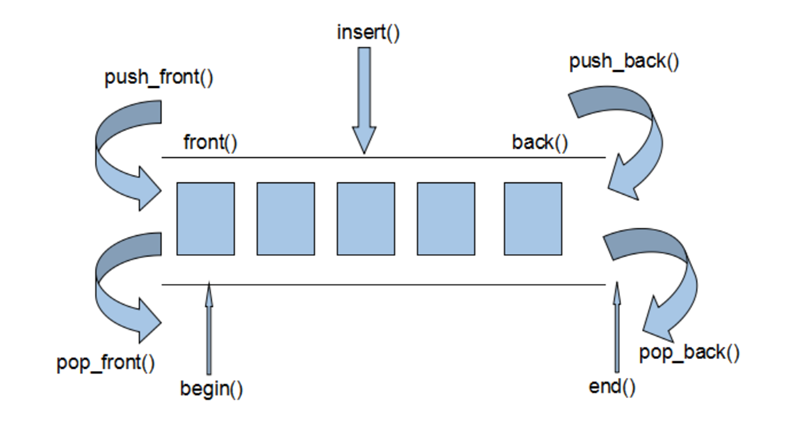Deque容器和vector容器最大的差异，一在于deque允许使用常数项时间对头端进行元素的插入和删除操作。二在于deque没有容量的概念，因为它是动态的以分段连续空间组合而成，随时可以增加一段新的空间并链接起来，换句话说，像vector那样，”旧空间不足而重新配置一块更大空间，然后复制元素，再释放旧空间”这样的事情在deque身上是不会发生的。也因此，deque没有必须要提供所谓的空间保留(reserve)功能.

### deque容器实现原理

Deque容器是连续的空间，至少逻辑上看来如此，连续现行空间总是令我们联想到array和vector,array无法成长，vector虽可成长，却只能向尾端成长，而且其成长其实是一个假象，事实上(1) 申请更大空间 (2)原数据复制新空间 (3)释放原空间 三步骤，如果不是vector每次配置新的空间时都留有余裕，其成长假象所带来的代价是非常昂贵的。

Deque是由一段一段的定量的连续空间构成。一旦有必要在deque前端或者尾端增加新的空间，便配置一段连续定量的空间，串接在deque的头端或者尾端。Deque最大的工作就是维护这些分段连续的内存空间的整体性的假象，并提供随机存取的接口，避开了重新配置空间，复制，释放的轮回，代价就是复杂的迭代器架构。

Deque采取一块所谓的map(注意，不是STL的map容器)作为主控，这里所谓的map是一小块连续的内存空间，其中每一个元素(此处成为一个结点)都是一个指针，指向另一段连续性内存空间，称作缓冲区。缓冲区才是deque的存储空间的主体。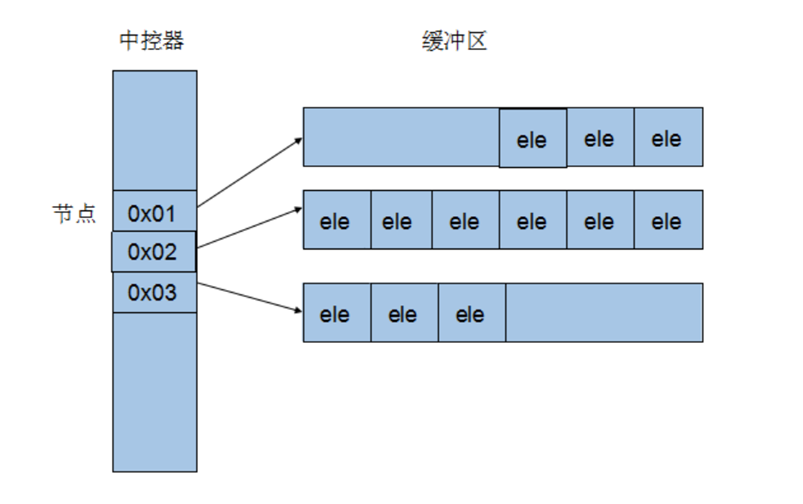### deque常用API

deque构造函数

deque<T> deqT;//默认构造形式
deque(beg, end);//构造函数将[beg, end)区间中的元素拷贝给本身。
deque(n, elem);//构造函数将n个elem拷贝给本身。
deque(const deque &deq);//拷贝构造函数。


deque赋值操作

assign(beg, end);//将[beg, end)区间中的数据拷贝赋值给本身。
assign(n, elem);//将n个elem拷贝赋值给本身。
deque& operator=(const deque &deq); //重载等号操作符
swap(deq);// 将deq与本身的元素互换


deque大小操作

deque.size();//返回容器中元素的个数
deque.empty();//判断容器是否为空
deque.resize(num);//重新指定容器的长度为num,若容器变长，则以默认值填充新位置。如果容器变短，则末尾超出容器长度的元素被删除。
deque.resize(num, elem); //重新指定容器的长度为num,若容器变长，则以elem值填充新位置,如果容器变短，则末尾超出容器长度的元素被删除。


deque双端插入和删除操作

push_back(elem);//在容器尾部添加一个数据
push_front(elem);//在容器头部插入一个数据
pop_back();//删除容器最后一个数据
pop_front();//删除容器第一个数据


deque数据存取

at(idx);//返回索引idx所指的数据，如果idx越界，抛出out_of_range。
operator[];//返回索引idx所指的数据，如果idx越界，不抛出异常，直接出错。
front();//返回第一个数据。
back();//返回最后一个数据


deque插入操作

insert(pos,elem);//在pos位置插入一个elem元素的拷贝，返回新数据的位置。
insert(pos,n,elem);//在pos位置插入n个elem数据，无返回值。
insert(pos,beg,end);//在pos位置插入[beg,end)区间的数据，无返回值。


deque删除操作

clear();//移除容器的所有数据
erase(beg,end);//删除[beg,end)区间的数据，返回下一个数据的位置。
erase(pos);//删除pos位置的数据，返回下一个数据的位置。


## 4. stack容器

### stack容器基本概念

stack是一种先进后出(First In Last Out,FILO)的数据结构，它只有一个出口，形式如图所示。stack容器允许新增元素，移除元素，取得栈顶元素，但是除了最顶端外，没有任何其他方法可以存取stack的其他元素。换言之，stack不允许有遍历行为。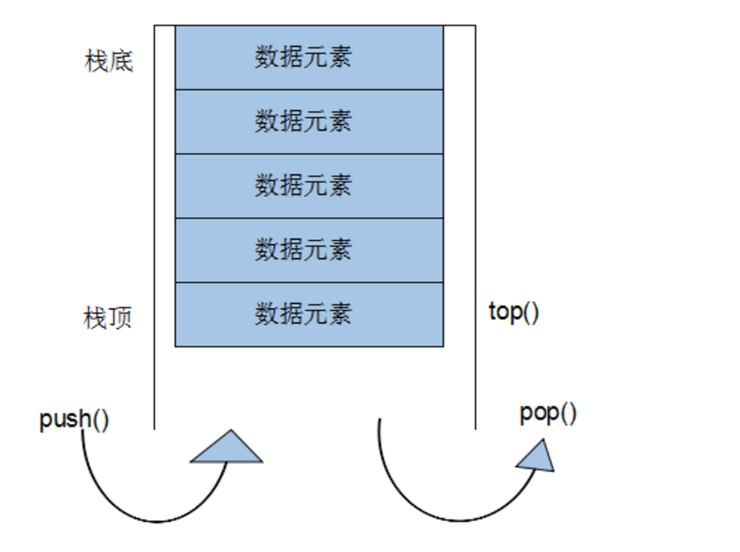### stack没有迭代器

Stack所有元素的进出都必须符合”先进后出”的条件，只有stack顶端的元素，才有机会被外界取用。Stack不提供遍历功能，也不提供迭代器。

### stack常用API

stack构造函数

stack<T> stkT;//stack采用模板类实现， stack对象的默认构造形式：
stack(const stack &stk);//拷贝构造函数


stack赋值操作

stack& operator=(const stack &stk);//重载等号操作符


stack数据存取操作

push(elem);//向栈顶添加元素
pop();//从栈顶移除第一个元素
top();//返回栈顶元素


stack大小操作

empty();//判断堆栈是否为空
size();//返回堆栈的大小


## 5. queue容器

### queue容器基本概念

Queue是一种先进先出(First In First Out,FIFO)的数据结构，它有两个出口，queue容器允许从一端新增元素，从另一端移除元素。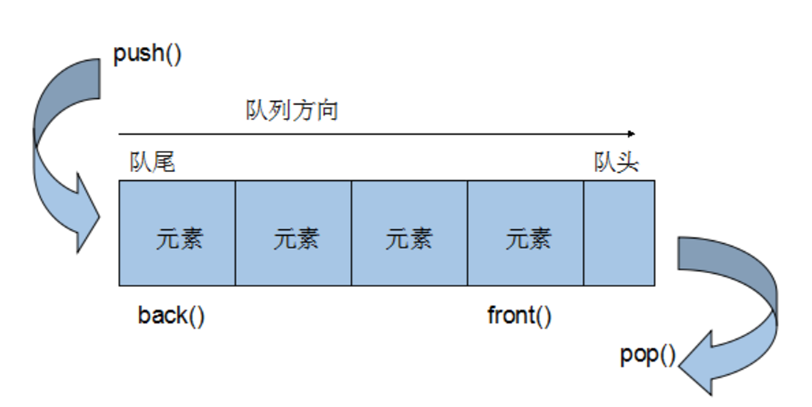### queue没有迭代器

Queue所有元素的进出都必须符合”先进先出”的条件，只有queue的顶端元素，才有机会被外界取用。Queue不提供遍历功能，也不提供迭代器。

### queue常用API

queue构造函数

queue<T> queT;//queue采用模板类实现，queue对象的默认构造形式：
queue(const queue &que);//拷贝构造函数


queue存取、插入和删除操作

push(elem);//往队尾添加元素
pop();//从队头移除第一个元素
back();//返回最后一个元素
front();//返回第一个元素


queue赋值操作

queue& operator=(const queue &que);//重载等号操作符


queue大小操作

empty();//判断队列是否为空
size();//返回队列的大小


## 6. list容器

list容器基本概念

List和vector是两个最常被使用的容器。

List容器是一个双向链表。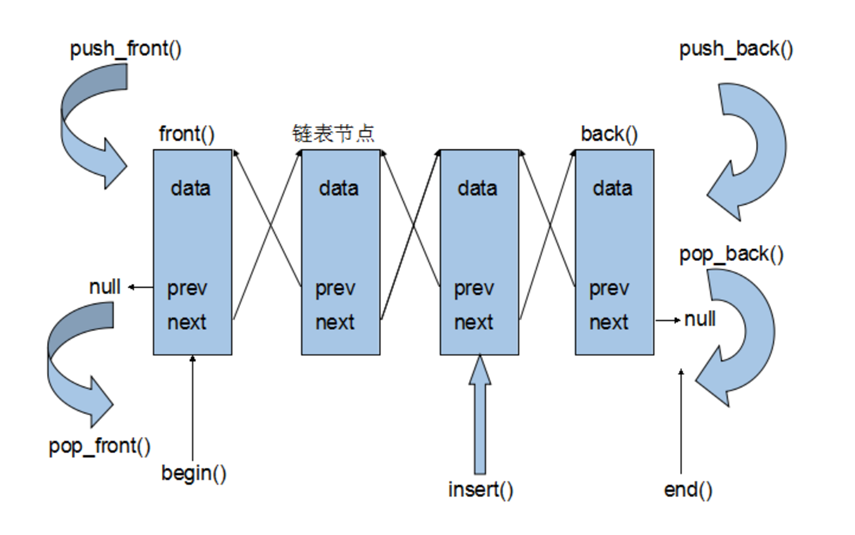• 采用动态存储分配，不会造成内存浪费和溢出
• 链表执行插入和删除操作十分方便，修改指针即可，不需要移动大量元素
• 链表灵活，但是空间和时间额外耗费较大

### list容器的迭代器

List容器不能像vector一样以普通指针作为迭代器，因为其节点不能保证在同一块连续的内存空间上。

List迭代器必须有能力指向list的节点，并有能力进行正确的递增、递减、取值、成员存取操作。所谓”list正确的递增，递减、取值、成员取用”是指，递增时指向下一个节点，递减时指向上一个节点，取值时取的是节点的数据值，成员取用时取的是节点的成员。

List有一个重要的性质，插入操作和删除操作都不会造成原有list迭代器的失效。这在vector是不成立的，因为vector的插入操作可能造成记忆体重新配置，导致原有的迭代器全部失效，甚至List元素的删除，也只有被删除的那个元素的迭代器失效，其他迭代器不受任何影响。

### list容器的数据结构

list容器不仅是一个双向链表，而且还是一个循环的双向链表。

#define _CRT_SECURE_NO_WARNINGS
#include<iostream>
#include<list>
using namespace std;

int main(){

list<int> myList;
for (int i = 0; i < 10; i ++){
myList.push_back(i);
}

for (int i = 0; i < myList._Mysize * 2;i++){
cout << "Node:" << node->_Myval << endl;
node = node->_Next;
node = node->_Next;
}
}

system("pause");
return EXIT_SUCCESS;
}


### list常用API

list构造函数

list<T> lstT;//list采用采用模板类实现,对象的默认构造形式：
list(beg,end);//构造函数将[beg, end)区间中的元素拷贝给本身。
list(n,elem);//构造函数将n个elem拷贝给本身。
list(const list &lst);//拷贝构造函数。


list数据元素插入和删除操作

push_back(elem);//在容器尾部加入一个元素
pop_back();//删除容器中最后一个元素
push_front(elem);//在容器开头插入一个元素
pop_front();//从容器开头移除第一个元素
insert(pos,elem);//在pos位置插elem元素的拷贝，返回新数据的位置。
insert(pos,n,elem);//在pos位置插入n个elem数据，无返回值。
insert(pos,beg,end);//在pos位置插入[beg,end)区间的数据，无返回值。
clear();//移除容器的所有数据
erase(beg,end);//删除[beg,end)区间的数据，返回下一个数据的位置。
erase(pos);//删除pos位置的数据，返回下一个数据的位置。
remove(elem);//删除容器中所有与elem值匹配的元素。


list大小操作

size();//返回容器中元素的个数
empty();//判断容器是否为空
resize(num);//重新指定容器的长度为num，

resize(num, elem);//重新指定容器的长度为num，



list赋值操作

assign(beg, end);//将[beg, end)区间中的数据拷贝赋值给本身。
assign(n, elem);//将n个elem拷贝赋值给本身。
list& operator=(const list &lst);//重载等号操作符
swap(lst);//将lst与本身的元素互换。


list数据的存取

front();//返回第一个元素。
back();//返回最后一个元素。


list反转排序

reverse();//反转链表，比如lst包含1,3,5元素，运行此方法后，lst就包含5,3,1元素。
sort(); //list排序


## 7. set/multiset容器

### set容器基本概念

Set的特性是。所有元素都会根据元素的键值自动被排序。Set的元素不像map那样可以同时拥有实值和键值，set的元素即是键值又是实值。Set不允许两个元素有相同的键值。

set拥有和list某些相同的性质，当对容器中的元素进行插入操作或者删除操作的时候，操作之前所有的迭代器，在操作完成之后依然有效，被删除的那个元素的迭代器必然是一个例外。

### multiset容器基本概念

multiset特性及用法和set完全相同，唯一的差别在于它允许键值重复。set和multiset的底层实现是红黑树.

### set常用API

set构造函数

set<T> st;//set默认构造函数：
mulitset<T> mst; //multiset默认构造函数:
set(const set &st);//拷贝构造函数


set赋值操作

set& operator=(const set &st);//重载等号操作符
swap(st);//交换两个集合容器


set大小操作

size();//返回容器中元素的数目
empty();//判断容器是否为空


set插入和删除操作

insert(elem);//在容器中插入元素。
clear();//清除所有元素
erase(pos);//删除pos迭代器所指的元素，返回下一个元素的迭代器。
erase(beg, end);//删除区间[beg,end)的所有元素 ，返回下一个元素的迭代器。
erase(elem);//删除容器中值为elem的元素。


set查找操作

find(key);//查找键key是否存在,若存在，返回该键的元素的迭代器；若不存在，返回set.end();
count(key);//查找键key的元素个数
lower_bound(keyElem);//返回第一个key>=keyElem元素的迭代器。
upper_bound(keyElem);//返回第一个key>keyElem元素的迭代器。
equal_range(keyElem);//返回容器中key与keyElem相等的上下限的两个迭代器。


set的返回值 指定set排序规则举例:

//插入操作返回值
void test01(){

set<int> s;
pair<set<int>::iterator,bool> ret = s.insert(10);
if (ret.second){
cout << "插入成功:" << *ret.first << endl;
}
else{
cout << "插入失败:" << *ret.first << endl;
}

ret = s.insert(10);
if(ret.second){
cout << "插入成功:" << *ret.first << endl;
}
else{
cout << "插入失败:" << *ret.first << endl;
}

}

struct MyCompare02{
bool operator()(int v1,int v2){
return v1 > v2;
}
};

//set从大到小
void test02(){

srand((unsigned int)time(NULL));
//我们发现set容器的第二个模板参数可以设置排序规则，默认规则是less<_Kty>
set<int, MyCompare02> s;
for (int i = 0; i < 10;i++){
s.insert(rand() % 100);
}

for (set<int, MyCompare02>::iterator it = s.begin(); it != s.end(); it ++){
cout << *it << " ";
}
cout << endl;
}

//set容器中存放对象
class Person{
public:
Person(string name,int age){
this->mName = name;
this->mAge = age;
}
public:
string mName;
int mAge;
};

struct MyCompare03{
bool operator()(const Person& p1,const Person& p2){
return p1.mAge > p2.mAge;
}
};

void test03(){

set<Person, MyCompare03> s;

Person p1("aaa", 20);
Person p2("bbb", 30);
Person p3("ccc", 40);
Person p4("ddd", 50);

s.insert(p1);
s.insert(p2);
s.insert(p3);
s.insert(p4);

for (set<Person, MyCompare03>::iterator it = s.begin(); it != s.end(); it++){
cout << "Name:" << it->mName << " Age:" << it->mAge << endl;
}

}


### 对组(pair)

//第一种方法创建一个对组
pair<string, int> pair1(string("name"), 20);
cout << pair1.first << endl; //访问pair第一个值
cout << pair1.second << endl;//访问pair第二个值
//第二种
pair<string, int> pair2 = make_pair("name", 30);
cout << pair2.first << endl;
cout << pair2.second << endl;
//pair=赋值
pair<string, int> pair3 = pair2;
cout << pair3.first << endl;
cout << pair3.second << endl;


## 8. map/multimap容器

### map/multimap基本概念

Map的特性是，所有元素都会根据元素的键值自动排序。

Map所有的元素都是pair,同时拥有实值和键值，pair的第一元素被视为键值，第二元素被视为实值，map不允许两个元素有相同的键值。

Map和list拥有相同的某些性质，当对它的容器元素进行新增操作或者删除操作时，操作之前的所有迭代器，在操作完成之后依然有效，当然被删除的那个元素的迭代器必然是个例外。

Multimap和map的操作类似，唯一区别multimap键值可重复。

Map和multimap都是以红黑树为底层实现机制。

### map/multimap常用API

map构造函数

map<T1, T2> mapTT;//map默认构造函数:
map(const map &mp);//拷贝构造函数


map赋值操作

map& operator=(const map &mp);//重载等号操作符
swap(mp);//交换两个集合容器


map大小操作

size();//返回容器中元素的数目
empty();//判断容器是否为空


map插入数据元素操作

map.insert(...); //往容器插入元素，返回pair<iterator,bool>
map<int, string> mapStu;
// 第一种 通过pair的方式插入对象
mapStu.insert(pair<int, string>(3, "小张"));
// 第二种 通过pair的方式插入对象
mapStu.inset(make_pair(-1, "校长"));
// 第三种 通过value_type的方式插入对象
mapStu.insert(map<int, string>::value_type(1, "小李"));
// 第四种 通过数组的方式插入值
mapStu = "小刘";
mapStu = "小王";


map删除操作

clear();//删除所有元素
erase(pos);//删除pos迭代器所指的元素，返回下一个元素的迭代器。
erase(beg,end);//删除区间[beg,end)的所有元素 ，返回下一个元素的迭代器。
erase(keyElem);//删除容器中key为keyElem的对组。


map查找操作

find(key);//查找键key是否存在,若存在，返回该键的元素的迭代器；/若不存在，返回map.end();
count(keyElem);//返回容器中key为keyElem的对组个数。对map来说，要么是0，要么是1。对multimap来说，值可能大于1。
lower_bound(keyElem);//返回第一个key>=keyElem元素的迭代器。
upper_bound(keyElem);//返回第一个key>keyElem元素的迭代器。
equal_range(keyElem);//返回容器中key与keyElem相等的上下限的两个迭代器。


multimap案例

//公司今天招聘了5个员工，5名员工进入公司之后，需要指派员工在那个部门工作
//人员信息有: 姓名 年龄 电话 工资等组成
//通过Multimap进行信息的插入 保存 显示
//分部门显示员工信息 显示全部员工信息

#define _CRT_SECURE_NO_WARNINGS

#include<iostream>
#include<map>
#include<string>
#include<vector>
using namespace std;

//multimap 案例
//公司今天招聘了 5 个员工，5 名员工进入公司之后，需要指派员工在那个部门工作
//人员信息有: 姓名 年龄 电话 工资等组成
//通过 Multimap 进行信息的插入 保存 显示
//分部门显示员工信息 显示全部员工信息

#define SALE_DEPATMENT 1 //销售部门
#define DEVELOP_DEPATMENT 2 //研发部门
#define FINACIAL_DEPATMENT 3 //财务部门
#define ALL_DEPATMENT 4 //所有部门

//员工类
class person{
public:
string name; //员工姓名
int age; //员工年龄
double salary; //员工工资
string tele; //员工电话
};

//创建5个员工
void CreatePerson(vector<person>& vlist){

string seed = "ABCDE";
for (int i = 0; i < 5; i++){
person p;
p.name = "员工";
p.name += seed[i];
p.age = rand() % 30 + 20;
p.salary = rand() % 20000 + 10000;
p.tele = "010-8888888";
vlist.push_back(p);
}

}

//5名员工分配到不同的部门
void PersonByGroup(vector<person>& vlist, multimap<int, person>& plist){

int operate = -1; //用户的操作

for (vector<person>::iterator it = vlist.begin(); it != vlist.end(); it++){

cout << "当前员工信息:" << endl;
cout << "姓名：" << it->name << " 年龄:" << it->age << " 工资:" << it->salary << " 电话：" << it->tele << endl;
cout << "请对该员工进行部门分配(1 销售部门, 2 研发部门, 3 财务部门):" << endl;
scanf("%d", &operate);

while (true){

if (operate == SALE_DEPATMENT){  //将该员工加入到销售部门
plist.insert(make_pair(SALE_DEPATMENT, *it));
break;
}
else if (operate == DEVELOP_DEPATMENT){
plist.insert(make_pair(DEVELOP_DEPATMENT, *it));
break;
}
else if (operate == FINACIAL_DEPATMENT){
plist.insert(make_pair(FINACIAL_DEPATMENT, *it));
break;
}
else{
cout << "您的输入有误，请重新输入(1 销售部门, 2 研发部门, 3 财务部门):" << endl;
scanf("%d", &operate);
}

}

}
cout << "员工部门分配完毕!" << endl;
cout << "***********************************************************" << endl;

}

//打印员工信息
void printList(multimap<int, person>& plist, int myoperate){

if (myoperate == ALL_DEPATMENT){
for (multimap<int, person>::iterator it = plist.begin(); it != plist.end(); it++){
cout << "姓名：" << it->second.name << " 年龄:" << it->second.age << " 工资:" << it->second.salary << " 电话：" << it->second.tele << endl;
}
return;
}

multimap<int, person>::iterator it = plist.find(myoperate);
int depatCount = plist.count(myoperate);
int num = 0;
if (it != plist.end()){
while (it != plist.end() && num < depatCount){
cout << "姓名：" << it->second.name << " 年龄:" << it->second.age << " 工资:" << it->second.salary << " 电话：" << it->second.tele << endl;
it++;
num++;
}
}
}

//根据用户操作显示不同部门的人员列表
void ShowPersonList(multimap<int, person>& plist, int myoperate){

switch (myoperate)
{
case SALE_DEPATMENT:
printList(plist, SALE_DEPATMENT);
break;
case DEVELOP_DEPATMENT:
printList(plist, DEVELOP_DEPATMENT);
break;
case FINACIAL_DEPATMENT:
printList(plist, FINACIAL_DEPATMENT);
break;
case ALL_DEPATMENT:
printList(plist, ALL_DEPATMENT);
break;
}
}

//用户操作菜单

int flag = -1;
int isexit = 0;
while (true){
cout << "请输入您的操作((1 销售部门, 2 研发部门, 3 财务部门, 4 所有部门, 0退出)：" << endl;
scanf("%d", &flag);

switch (flag)
{
case SALE_DEPATMENT:
ShowPersonList(plist, SALE_DEPATMENT);
break;
case DEVELOP_DEPATMENT:
ShowPersonList(plist, DEVELOP_DEPATMENT);
break;
case FINACIAL_DEPATMENT:
ShowPersonList(plist, FINACIAL_DEPATMENT);
break;
case ALL_DEPATMENT:
ShowPersonList(plist, ALL_DEPATMENT);
break;
case 0:
isexit = 1;
break;
default:
cout << "您的输入有误，请重新输入!" << endl;
break;
}

if (isexit == 1){
break;
}
}

}

int main(){

vector<person>  vlist; //创建的5个员工 未分组
multimap<int, person> plist; //保存分组后员工信息

//创建5个员工
CreatePerson(vlist);
//5名员工分配到不同的部门
PersonByGroup(vlist, plist);
//根据用户输入显示不同部门员工信息列表 或者 显示全部员工的信息列表

system("pause");
return EXIT_SUCCESS;
}


## STL容器使用时机

.vectordequelistsetmultisetmapmultimap

vector的使用场景：比如软件历史操作记录的存储，我们经常要查看历史记录，比如上一次的记录，上上次的记录，但却不会去删除记录，因为记录是事实的描述。

deque的使用场景：比如排队购票系统，对排队者的存储可以采用deque，支持头端的快速移除，尾端的快速添加。如果采用vector，则头端移除时，会移动大量的数据，速度慢。

vector与deque的比较：

list的使用场景：比如公交车乘客的存储，随时可能有乘客下车，支持频繁的不确实位置元素的移除插入。

set的使用场景：比如对手机游戏的个人得分记录的存储，存储要求从高分到低分的顺序排列。

map的使用场景：比如按ID号存储十万个用户，想要快速要通过ID查找对应的用户。二叉树的查找效率，这时就体现出来了。如果是vector容器，最坏的情况下可能要遍历完整个容器才能找到该用户。

# 常用算法

## 1. 函数对象

1. 函数对象(仿函数)是一个类，不是一个函数。
2. 函数对象(仿函数)重载了”() ”操作符使得它可以像函数一样调用。

STL提供的算法往往都有两个版本，其中一个版本表现出最常用的某种运算，另一版本则允许用户通过template参数的形式来指定所要采取的策略。

//函数对象是重载了函数调用符号的类
class MyPrint
{
public:
MyPrint()
{
m_Num = 0;
}
int m_Num;

public:
void operator() (int num)
{
cout << num << endl;
m_Num++;
}
};

//函数对象
//重载了()操作符的类实例化的对象，可以像普通函数那样调用,可以有参数 ，可以有返回值
void test01()
{
MyPrint myPrint;
myPrint(20);

}
// 函数对象超出了普通函数的概念，可以保存函数的调用状态
void test02()
{
MyPrint myPrint;
myPrint(20);
myPrint(20);
myPrint(20);
cout << myPrint.m_Num << endl;
}

{
print(num);
}

//函数对象作为参数
void test03()
{
//参数1：匿名函数对象
}


1、函数对象通常不定义构造函数和析构函数，所以在构造和析构时不会发生任何问题，避免了函数调用的运行时问题。
2、函数对象超出普通函数的概念，函数对象可以有自己的状态
3、函数对象可内联编译，性能好。用函数指针几乎不可能
4、模版函数对象使函数对象具有通用性，这也是它的优势之一

## 2. 谓词

class GreaterThenFive
{
public:
bool operator()(int num)
{
return num > 5;
}

};
//一元谓词
void test01()
{
vector<int> v;
for (int i = 0; i < 10;i ++)
{
v.push_back(i);
}

vector<int>::iterator it =  find_if(v.begin(), v.end(), GreaterThenFive());
if (it == v.end())
{
cout << "没有找到" << endl;
}
else
{
cout << "找到了: " << *it << endl;
}
}

//二元谓词
class MyCompare
{
public:
bool operator()(int num1, int num2)
{
return num1 > num2;
}
};

void test02()
{
vector<int> v;
v.push_back(10);
v.push_back(40);
v.push_back(20);
v.push_back(90);
v.push_back(60);

//默认从小到大
sort(v.begin(), v.end());
for (vector<int>::iterator it = v.begin(); it != v.end();it++)
{
cout << *it << " ";
}
cout << endl;
cout << "----------------------------" << endl;
//使用函数对象改变算法策略，排序从大到小
sort(v.begin(), v.end(),MyCompare());
for (vector<int>::iterator it = v.begin(); it != v.end(); it++)
{
cout << *it << " ";
}
cout << endl;
}


## 3.内建函数对象

STL内建了一些函数对象。分为:算数类函数对象,关系运算类函数对象，逻辑运算类仿函数。这些仿函数所产生的对象，用法和一般函数完全相同，当然我们还可以产生无名的临时对象来履行函数功能。使用内建函数对象，需要引入头文件

#include<functional>。


6个算数类函数对象,除了negate是一元运算，其他都是二元运算。

template<class T> T plus<T>//加法仿函数
template<class T> T minus<T>//减法仿函数
template<class T> T multiplies<T>//乘法仿函数
template<class T> T divides<T>//除法仿函数
template<class T> T modulus<T>//取模仿函数
template<class T> T negate<T>//取反仿函数


6个关系运算类函数对象,每一种都是二元运算。

template<class T> bool equal_to<T>//等于
template<class T> bool not_equal_to<T>//不等于
template<class T> bool greater<T>//大于
template<class T> bool greater_equal<T>//大于等于
template<class T> bool less<T>//小于
template<class T> bool less_equal<T>//小于等于


template<class T> bool logical_and<T>//逻辑与
template<class T> bool logical_or<T>//逻辑或
template<class T> bool logical_not<T>//逻辑非


//取反仿函数
void test01()
{
negate<int> n;
cout << n(50) << endl;
}

//加法仿函数
void test02()
{
plus<int> p;
cout << p(10, 20) << endl;
}

//大于仿函数
void test03()
{
vector<int> v;
srand((unsigned int)time(NULL));
for (int i = 0; i < 10; i++){
v.push_back(rand() % 100);
}

for (vector<int>::iterator it = v.begin(); it != v.end(); it++){
cout << *it << " ";
}
cout << endl;
sort(v.begin(), v.end(), greater<int>());

for (vector<int>::iterator it = v.begin(); it != v.end(); it++){
cout << *it << " ";
}
cout << endl;

}


## 4. 函数对象适配器

//函数适配器bind1st bind2nd
//现在我有这个需求 在遍历容器的时候，我希望将容器中的值全部加上100之后显示出来，怎么做？
//我们直接给函数对象绑定参数 编译阶段就会报错
//for_each(v.begin(), v.end(), bind2nd(myprint(),100));
//如果我们想使用绑定适配器,需要我们自己的函数对象继承binary_function 或者 unary_function
//根据我们函数对象是一元函数对象 还是二元函数对象
class MyPrint :public binary_function<int,int,void>
{
public:
void operator()(int v1,int v2) const
{
cout << "v1 = : " << v1 << " v2 = :" <<v2  << " v1+v2 = :" << (v1 + v2) << endl;
}
};
//1、函数适配器
void test01()
{
vector<int>v;
for (int i = 0; i < 10; i++)
{
v.push_back(i);
}
cout << "请输入起始值：" << endl;
int x;
cin >> x;

for_each(v.begin(), v.end(), bind1st(MyPrint(), x));
//for_each(v.begin(), v.end(), bind2nd( MyPrint(),x ));
}
//总结：  bind1st和bind2nd区别?
//bind1st ： 将参数绑定为函数对象的第一个参数
//bind2nd ： 将参数绑定为函数对象的第二个参数
//bind1st bind2nd将二元函数对象转为一元函数对象

class GreaterThenFive:public unary_function<int,bool>
{
public:
bool operator ()(int v) const
{
return v > 5;
}
};

//2、取反适配器
void test02()
{
vector <int> v;
for (int i = 0; i < 10;i++)
{
v.push_back(i);
}

// 	vector<int>::iterator it =  find_if(v.begin(), v.end(), GreaterThenFive()); //返回第一个大于5的迭代器
//	vector<int>::iterator it = find_if(v.begin(), v.end(),  not1(GreaterThenFive())); //返回第一个小于5迭代器
//自定义输入
vector<int>::iterator it = find_if(v.begin(), v.end(), not1 ( bind2nd(greater<int>(),5)));
if (it == v.end())
{
cout << "没找到" << endl;
}
else
{
cout << "找到" << *it << endl;
}

//排序  二元函数对象
sort(v.begin(), v.end(), not2(less<int>()));
for_each(v.begin(), v.end(), [](int val){cout << val << " "; });

}
//not1 对一元函数对象取反
//not2 对二元函数对象取反

void MyPrint03(int v,int v2)
{
cout << v + v2<< " ";
}

//3、函数指针适配器   ptr_fun
void test03()
{
vector <int> v;
for (int i = 0; i < 10; i++)
{
v.push_back(i);
}
// ptr_fun( )把一个普通的函数指针适配成函数对象
for_each(v.begin(), v.end(), bind2nd( ptr_fun( MyPrint03 ), 100));
}

//4、成员函数适配器
class Person
{
public:
Person(string name, int age)
{
m_Name = name;
m_Age = age;
}

//打印函数
void ShowPerson(){
cout << "成员函数:" << "Name:" << m_Name << " Age:" << m_Age << endl;
}
void Plus100()
{
m_Age += 100;
}
public:
string m_Name;
int m_Age;
};

void MyPrint04(Person &p)
{
cout << "姓名：" <<  p.m_Name << " 年龄：" << p.m_Age << endl;

};

void test04()
{
vector <Person>v;
Person p1("aaa", 10);
Person p2("bbb", 20);
Person p3("ccc", 30);
Person p4("ddd", 40);
v.push_back(p1);
v.push_back(p2);
v.push_back(p3);
v.push_back(p4);

//for_each(v.begin(), v.end(), MyPrint04);
//利用 mem_fun_ref 将Person内部成员函数适配
for_each(v.begin(), v.end(), mem_fun_ref(&Person::ShowPerson));
// 	for_each(v.begin(), v.end(), mem_fun_ref(&Person::Plus100));
// 	for_each(v.begin(), v.end(), mem_fun_ref(&Person::ShowPerson));
}

void test05(){

vector<Person*> v1;
//创建数据
Person p1("aaa", 10);
Person p2("bbb", 20);
Person p3("ccc", 30);
Person p4("ddd", 40);

v1.push_back(&p1);
v1.push_back(&p2);
v1.push_back(&p3);
v1.push_back(&p4);

for_each(v1.begin(), v1.end(), mem_fun(&Person::ShowPerson));
}

//如果容器存放的是对象指针，  那么用mem_fun
//如果容器中存放的是对象实体，那么用mem_fun_ref


## 算法概述

<algorithm>是所有STL头文件中最大的一个,其中常用的功能涉及到比较，交换，查找,遍历，复制，修改，反转，排序，合并等…
<numeric>体积很小，只包括在几个序列容器上进行的简单运算的模板函数.
<functional> 定义了一些模板类,用以声明函数对象。

### 1. 常用遍历算法

/*
遍历算法 遍历容器元素
@param beg 开始迭代器
@param end 结束迭代器
@param _callback  函数回调或者函数对象
@return 函数对象
*/
for_each(iterator beg, iterator end, _callback);
/*
transform算法 将指定容器区间元素搬运到另一容器中
注意 : transform 不会给目标容器分配内存，所以需要我们提前分配好内存
@param beg1 源容器开始迭代器
@param end1 源容器结束迭代器
@param beg2 目标容器开始迭代器
@param _cakkback 回调函数或者函数对象
@return 返回目标容器迭代器
*/
transform(iterator beg1, iterator end1, iterator beg2, _callbakc)

for_each:
/*

template<class _InIt,class _Fn1> inline
void for_each(_InIt _First, _InIt _Last, _Fn1 _Func)
{
for (; _First != _Last; ++_First)
_Func(*_First);
}

*/

//普通函数
void print01(int val){
cout << val << " ";
}
//函数对象
struct print001{
void operator()(int val){
cout << val << " ";
}
};

//for_each算法基本用法
void test01(){

vector<int> v;
for (int i = 0; i < 10;i++){
v.push_back(i);
}

//遍历算法
for_each(v.begin(), v.end(), print01);
cout << endl;

for_each(v.begin(), v.end(), print001());
cout << endl;

}

struct print02{
print02(){
mCount = 0;
}
void operator()(int val){
cout << val << " ";
mCount++;
}
int mCount;
};

//for_each返回值
void test02(){

vector<int> v;
for (int i = 0; i < 10; i++){
v.push_back(i);
}

print02 p = for_each(v.begin(), v.end(), print02());
cout << endl;
cout << p.mCount << endl;
}

struct print03 : public binary_function<int, int, void>{
void operator()(int val,int bindParam) const{
cout << val + bindParam << " ";
}
};

//for_each绑定参数输出
void test03(){

vector<int> v;
for (int i = 0; i < 10; i++){
v.push_back(i);
}

for_each(v.begin(), v.end(), bind2nd(print03(),100));
}

transform:
//transform 将一个容器中的值搬运到另一个容器中
/*
template<class _InIt, class _OutIt, class _Fn1> inline
_OutIt _Transform(_InIt _First, _InIt _Last,_OutIt _Dest, _Fn1 _Func)
{

for (; _First != _Last; ++_First, ++_Dest)
*_Dest = _Func(*_First);
return (_Dest);
}

template<class _InIt1,class _InIt2,class _OutIt,class _Fn2> inline
_OutIt _Transform(_InIt1 _First1, _InIt1 _Last1,_InIt2 _First2, _OutIt _Dest, _Fn2 _Func)
{
for (; _First1 != _Last1; ++_First1, ++_First2, ++_Dest)
*_Dest = _Func(*_First1, *_First2);
return (_Dest);
}
*/

struct transformTest01{
int operator()(int val){
return val + 100;
}
};
struct print01{
void operator()(int val){
cout << val << " ";
}
};
void test01(){

vector<int> vSource;
for (int i = 0; i < 10;i ++){
vSource.push_back(i + 1);
}

//目标容器
vector<int> vTarget;
//给vTarget开辟空间
vTarget.resize(vSource.size());
//将vSource中的元素搬运到vTarget
vector<int>::iterator it = transform(vSource.begin(), vSource.end(), vTarget.begin(), transformTest01());
//打印
for_each(vTarget.begin(), vTarget.end(), print01()); cout << endl;

}

//将容器1和容器2中的元素相加放入到第三个容器中
struct transformTest02{
int operator()(int v1,int v2){
return v1 + v2;
}
};
void test02(){

vector<int> vSource1;
vector<int> vSource2;
for (int i = 0; i < 10; i++){
vSource1.push_back(i + 1);
}

//目标容器
vector<int> vTarget;
//给vTarget开辟空间
vTarget.resize(vSource1.size());
transform(vSource1.begin(), vSource1.end(), vSource2.begin(),vTarget.begin(), transformTest02());
//打印
for_each(vTarget.begin(), vTarget.end(), print01()); cout << endl;
}


### 2. 常用查找算法

/*
find算法 查找元素
@param beg 容器开始迭代器
@param end 容器结束迭代器
@param value 查找的元素
@return 返回查找元素的位置
*/
find(iterator beg, iterator end, value)
/*
find_if算法 条件查找
@param beg 容器开始迭代器
@param end 容器结束迭代器
@param  callback 回调函数或者谓词(返回bool类型的函数对象)
@return bool 查找返回true 否则false
*/
find_if(iterator beg, iterator end, _callback);

/*
@param beg 容器开始迭代器
@param end 容器结束迭代器
@param  _callback 回调函数或者谓词(返回bool类型的函数对象)
@return 返回相邻元素的第一个位置的迭代器
*/
/*
binary_search算法 二分查找法
注意: 在无序序列中不可用
@param beg 容器开始迭代器
@param end 容器结束迭代器
@param value 查找的元素
@return bool 查找返回true 否则false
*/
bool binary_search(iterator beg, iterator end, value);
/*
count算法 统计元素出现次数
@param beg 容器开始迭代器
@param end 容器结束迭代器
@param  value回调函数或者谓词(返回bool类型的函数对象)
@return int返回元素个数
*/
count(iterator beg, iterator end, value);
/*
count算法 统计元素出现次数
@param beg 容器开始迭代器
@param end 容器结束迭代器
@param  callback 回调函数或者谓词(返回bool类型的函数对象)
@return int返回元素个数
*/
count_if(iterator beg, iterator end, _callback);


### 3. 常用排序算法

/*
merge算法 容器元素合并，并存储到另一容器中
@param beg1 容器1开始迭代器
@param end1 容器1结束迭代器
@param beg2 容器2开始迭代器
@param end2 容器2结束迭代器
@param dest  目标容器开始迭代器
*/
merge(iterator beg1, iterator end1, iterator beg2, iterator end2, iterator dest)
/*
sort算法 容器元素排序
注意:两个容器必须是有序的
@param beg 容器1开始迭代器
@param end 容器1结束迭代器
@param _callback 回调函数或者谓词(返回bool类型的函数对象)
*/
sort(iterator beg, iterator end, _callback)
/*
sort算法 对指定范围内的元素随机调整次序
@param beg 容器开始迭代器
@param end 容器结束迭代器
*/
random_shuffle(iterator beg, iterator end)
/*
reverse算法 反转指定范围的元素
@param beg 容器开始迭代器
@param end 容器结束迭代器
*/
reverse(iterator beg, iterator end)


### 4. 常用拷贝和替换算法

/*
copy算法 将容器内指定范围的元素拷贝到另一容器中
@param beg 容器开始迭代器
@param end 容器结束迭代器
@param dest 目标起始迭代器
*/
copy(iterator beg, iterator end, iterator dest)
/*
replace算法 将容器内指定范围的旧元素修改为新元素
@param beg 容器开始迭代器
@param end 容器结束迭代器
@param oldvalue 旧元素
@param oldvalue 新元素
*/
replace(iterator beg, iterator end, oldvalue, newvalue)
/*
replace_if算法 将容器内指定范围满足条件的元素替换为新元素
@param beg 容器开始迭代器
@param end 容器结束迭代器
@param callback函数回调或者谓词(返回Bool类型的函数对象)
@param oldvalue 新元素
*/
replace_if(iterator beg, iterator end, _callback, newvalue)
/*
swap算法 互换两个容器的元素
@param c1容器1
@param c2容器2
*/
swap(container c1, container c2)


### 5. 常用算数生成算法

/*
accumulate算法 计算容器元素累计总和
@param beg 容器开始迭代器
@param end 容器结束迭代器
@param value累加值
*/
accumulate(iterator beg, iterator end, value)
/*
fill算法 向容器中添加元素
@param beg 容器开始迭代器
@param end 容器结束迭代器
@param value t填充元素
*/
fill(iterator beg, iterator end, value)


### 6. 常用集合算法

/*
set_intersection算法 求两个set集合的交集
注意:两个集合必须是有序序列
@param beg1 容器1开始迭代器
@param end1 容器1结束迭代器
@param beg2 容器2开始迭代器
@param end2 容器2结束迭代器
@param dest  目标容器开始迭代器
@return 目标容器的最后一个元素的迭代器地址
*/
set_intersection(iterator beg1, iterator end1, iterator beg2, iterator end2, iterator dest)
/*
set_union算法 求两个set集合的并集
注意:两个集合必须是有序序列
@param beg1 容器1开始迭代器
@param end1 容器1结束迭代器
@param beg2 容器2开始迭代器
@param end2 容器2结束迭代器
@param dest  目标容器开始迭代器
@return 目标容器的最后一个元素的迭代器地址
*/
set_union(iterator beg1, iterator end1, iterator beg2, iterator end2, iterator dest)
/*
set_difference算法 求两个set集合的差集
注意:两个集合必须是有序序列
@param beg1 容器1开始迭代器
@param end1 容器1结束迭代器
@param beg2 容器2开始迭代器
@param end2 容器2结束迭代器
@param dest  目标容器开始迭代器
@return 目标容器的最后一个元素的迭代器地址
*/
set_difference(iterator beg1, iterator end1, iterator beg2, iterator end2, iterator dest)


02-241万+
01-07
03-24
08-2483
05-11
01-31920
03-05380
03-11282
01-1111万+
08-2115万+
01-24973
11-02185
03-2255
02-244226
07-267251
10-24212
04-04828
07-27198
07-212889
07-026963
11-27433

### “相关推荐”对你有帮助么？

•非常没帮助
•没帮助
•一般
•有帮助
•非常有帮助被折叠的  条评论 为什么被折叠?到【灌水乐园】发言¥2 ¥4 ¥6 ¥10 ¥20余额支付 (余额：-- )扫码支付获取中扫码支付点击重新获取扫码支付1.余额是钱包充值的虚拟货币，按照1:1的比例进行支付金额的抵扣。
2.余额无法直接购买下载，可以购买VIP、C币套餐、付费专栏及课程。余额充值